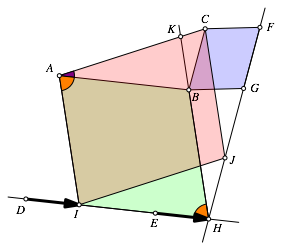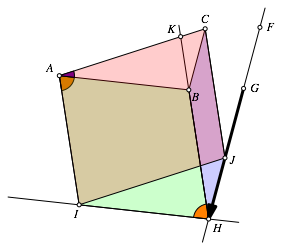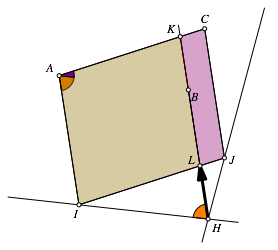Hans Walser, 

Generalization of Pythagoras

# 1     Problem

“In the triangle ABC let any parallelograms ABED, BCFG be drawn on AB, AC and let DE, FG meet in H. Join HB and produce it to meet AC in K. The sum of the parallelograms ABED, BCFG can then be shown to the equal to the parallelogram contained by AC, HB in an angle equal to the sum of the angles BAC, DHB.” [1, Book IV]Fig. 0: Red = Green + Blue

# 2     Proof without words

(using the principle of Cavalieri)Fig. 1: First stepFig. 2: Second stepFig. 3: Third step

References